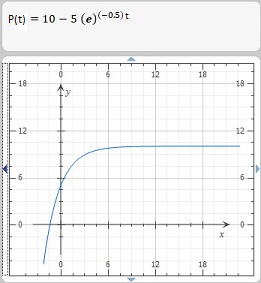# Psychologists interested in learning theory study learning curves. A learning curve is the graph...

## Question:

Psychologists interested in learning theory study learning curves. A learning curve is the graph of a function P(t), the performance of someone learning a skill as a function of the training time t. The derivative {eq}\frac{dP}{dt} {/eq} represents the rate at which performance improves.

(a) When do you think P increases most rapidly? What happens to {eq}\frac{dP}{dt} {/eq} as t increases? Explain.

(b) If M is the maximum level of performance of which the learner is capable, explain why the differential equation

{eq}\frac{dP}{dt} = k(M - P) {/eq} k a positive constant

is a reasonable model for learning.

(c) Make a rough sketch of a possible solution of this differential equation.

## Differential Equations, Exponential and Logarithmic Functions:

The exponential function is used in many real life applications as a mathematical model for the exponential growth or decay of an entity. Starting from an initial value problem describing the rate of change of a quantity, usually with respect to the time variable, as a differential equation, we arrive at an exponential function as the solution to the problem. The solution provides the state of the quantity in question, for any value of the time variable.

The exponential function in a solution models growth or decay based on the constant in the exponential power. Positive constants imply growth and negative constants imply decay.

(a) When do you think P increases most rapidly? What happens to {eq}\frac{dP}{dt} {/eq} as t increases?

The derivative {eq}\frac{dP}{dt} {/eq} represents the rate at which performance improves.

Supposing the learner starts with a score of 5 out of 10 in a performance test, 10 being the maximum possible score. Supposing the learner is tested immediately following a good training that focuses on improving the knowledge and performance based on the subject being taught. This is probably the time when the rate of change in performance would be most rapid, during times fairly close to the end of the training period.

The rate of improvement of performance would most probably level off as time passes, due to the learner not using the skills learned or due to memory storage being filled up by other needs, as is usual. However consistent use of the learned skill and continuous training of required or newer skills could keep the performance rate from leveling off.

(b) If M is the maximum level of performance of which the learner is capable, explain why the differential equation

{eq}\frac{dP}{dt} = k(M - P) {/eq} k a positive constant

is a reasonable model for learning.

The above differential equation states that the rate of change of performance is proportional to the difference between maximum performance and the current performance level. This is reasonable since the farther the current performance level is from maximum performance scores, the greater the rate of change in performance, provided that the expectancy of the training involved and goal of the learner, is to achieve maximum performance.

(c) Make a rough sketch of a possible solution of this differential equation.

We apply integration using separation of variables on the given differential equation in (b), to find a solution to the problem. We are not given any initial conditions, hence we set up the condition that the performance of the learner at time {eq}t = 0 {/eq} is {eq}P(0) {/eq}.

{eq}\begin{align*} \frac{dP}{dt} &= k(M - P) \\ \frac{dP}{M - P} &= k\\ \frac{dP}{P - M} &= - k \\ \int \frac{1}{P - M} \; dP &= \int -k \; dt \\ \ln ( P - M ) &= - kt + C_1\\ e^{ \ln ( P - M) } &= e^{-kt} \times e^{C_1} \\ P - M &= Ce^{-kt} \; &\text{(1)} \end{align*} {/eq}

We must solve for the integration constant {eq}C {/eq} that we introduced. We apply the initial condition {eq}P(0) {/eq} at {eq}t = 0 {/eq} to equation (1).

We get,

{eq}\begin{align*} P(0) - M &= Ce^{0} \\ P(0) - M &= C \\ \end{align*} {/eq}

Substituting the value of the above integration constant in equation (1) we get,

{eq}\begin{align*} P - M &= (P(0) - M)e^{-kt} \\ P(t) &= M + (P(0) - M)e^{-kt} \; &\text{(2)} \end{align*} {/eq}

Equation (2) is a solution for the given differential equation in (b).

To sketch the solution, suppose we assume that, {eq}M = 10, \; P(0) = 5, \; k = \frac{1}{2} > 0 {/eq}

The following graph is a sample learning curve of the above solution for the given model differential equation in (b).